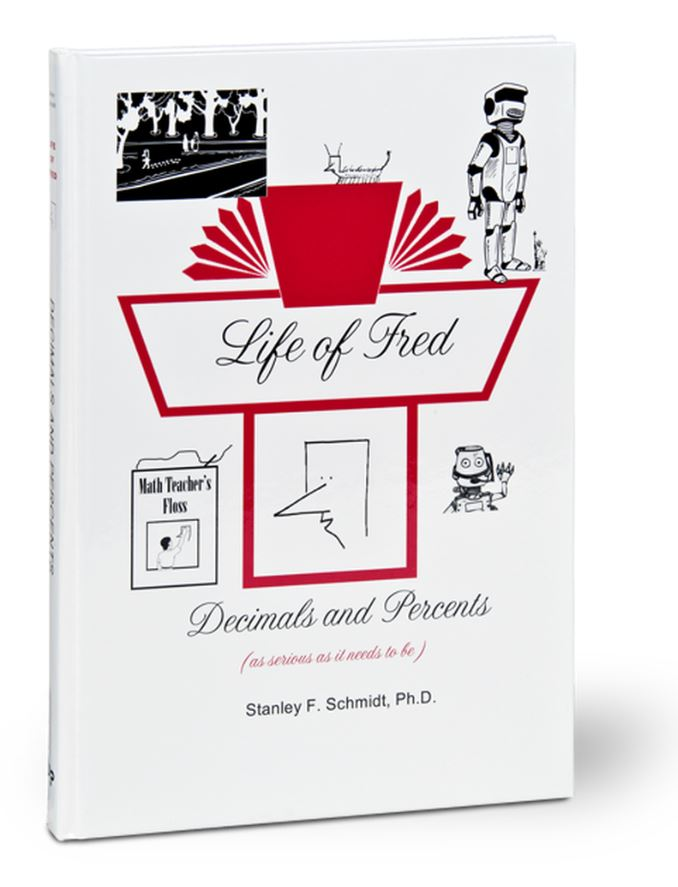# Life of Fred Middle School Guide book Set

\$ 125.00

This set can be started with any child who can add and subtract well and knows how to do long multiplication and division.  This set would be considered a middle school math program, for students in 5th through 9th grades. When they are finished these books, they are ready to begin the High School Set. Many high school aged students would benefit by going quickly through these books to lay foundations that they might have missed.

This set includes:

Life of Fred: Fractions

Life of Fred: Decimals and Percents

Life of Fred: Pre-Algebra 0 with Physics

Life of Fred: Pre-Algebra 1 with Biology

Life of Fred: Pre-Algebra 2 with Economics

Life of Fred: Fractions (Sample Pages)

This book lays the first half of the foundation for pre-algebra including . . .

• Less Than
• Billion
• Cardinal and Ordinal Numbers
• Savings and Expenses
• Definition of a Fraction
• Sectors
• Comparing Fractions
• Reducing Fractions
• Common Denominators
• Roman Numerals
• Least Common Multiples
• Improper Fractions
• Lines of Symmetry
• Division by Zero
• Circumference
• Multiplying Mixed Numbers
• Commutative Law
• Canceling
• Definition of a Function
• Area
• Unit Analysis
• Division of Fractions
• Geometric Figures
• and much more . . .

Life of Fred: Decimals and Percents (sample pages)

This book covers second half of the introduction to pre-algebra including . . .

• Number Systems
• Multiplying by Ten
• Functions and Inverse Functions
• Pi
• Multiplying Decimals
• Whole Numbers
• Squaring a Number
• Subtracting Mixed Units
• Sets and Subsets
• Union and Intersection of Sets
• Rules of Divisibility
• Division of Decimals
• Repeating Decimals
• Bar Graphs
• Prime and Composite Numbers
• Consecutive Numbers
• The Goldbach Conjecture
• Area of a Circle
• Dollars vs. Cents
• Pie Charts
• Conversion between Percents/ Fractions/Decimals
• 40% of 15
• 30% off
• Distance Equals Rate Times Time
• 15% more
• Area of a Triangle
• Square Roots
• Area of a Parallelogram
• 13 Is What Percent of 52
• Ratio
• Ordered Pairs
• Graphing
• Negative Numbers
• Elapsed Time
• Probability
• And more . . .

Life of Fred: Pre-Algebra 0 with Physics (Sample Pages)

elementary physics:

• Numerals
• Making models
• Nineteen conversion factor problems
• Area of a rectangle
• Friction independent of speed
• Constants of Proportionality
• Exact speed of light
• Continuous and discrete variables
• Story of the meter
• Pendulums
• Oblate spheroids
• Square roots
• Normal forces
• Coefficient of friction
• Solving d = rt for r
• Graphing points
• Ordered pairs
• Why rocks stop falling
• Hunch-conjecture-theory-law
• Simultaneity doesn't exist
• Inductive and deductive reasoning
• How fossils and astronomy are connected
• Hooke's law
• Three meanings of plastic
• Static vs. kinetic friction
• Nine forms of energy
• Energy Cards game
• Experimentally finding the coefficient of static friction without knowing the weight of the object
• Resolving a vector into its components
• Similar triangles
• Slope
• Physicists think that there isn't any work in holding a bowling ball
• Kelvin temperature
• Law of conservation of energy
• Perpetual motion machines
• Kilowatt-hours
• Photosynthesis
• Metric system
• Gregorian calendar
• Two ways to measure mass
• Definition of pi
• Inertia
• Newton's first and second laws
• Definition of pressure
• Exponents
• Density of neutron stars
• Computing the mass of an iron atom
• Cavalieri's principle
• Pressure at a given depth
• Buoyancy
• Finding the volume of a desk lamp
• Air has mass
• Why 35-foot straws don't work
• Gedankenexperiments
• Elliptical constructions
• Galvani and dead frogs that twitch
• Schematic drawings in electrical circuits
• Electrons per second in an ampere
• Ohm's law
• Batteries in series
• Computing resistance in parallel circuits
• Dangers of electricity—Thomas Merton
• History of the atom
• Principal quantum number
• Orbital quantum number
• Orbital magnetic quantum number
• Spin magnetic quantum number
• Positron

Life of Fred: Pre-Algebra 1 with Biology (Sample Pages)

including

• Definition of Life
• Sets
• Fractions
• Germination of Seeds
• Area of a Rectangle
• Volume of a Cube
• Ordinal Numbers
• Diameter and Circumference of a Circle
• Definition of ?
• 2% of 500
• Four Ways Plants Make New Plants
• d = rt
• 20% Discount
• The Five Kingdoms
• Phyla
• Classes
• Orders
• Families
• Genera
• Species
• Conversion Factors
• Where the Non-Water Mass of a Plant Comes From — Plants Don't Eat Dirt
• Subsets of Sets
• Digestion
• Eyes
• Negative Numbers
• Dominant Genes
• Genotypes
• Phenotypes
• Blood
• Staying Alive
• Solving Algebraic Equations
• Volume of a Cylinder
• Word Problems
• Breathing
• Chlorophyll vs. Hemoglobin vs. Hemocyanin
• Stoichiometry
• The Whole Numbers
• A Proof that Division by Zero is Not Permitted
• Bones
• The Integumentary System
• Epidermis and Dermis
• Meiosis and Mitosis
• Chromosomes
• DNA
• Alleles

Life of Fred: Pre-Algebra 2 with Economics (Sample Pages)

including

• Domain and codomain of a function
• Conversion factors
• Steps in Solving Word Problems
• One-to-one Functions, Unit Analysis
• Key to a Successful Business
• Five Qualities that Money Should Have
• Definitions of Capitalism, Socialism, and Communism
• Payday Loans
• Interest Rates
• Partnerships
• Cardinality of a Set
• Four Ways to Kill Competition
• Freedom vs. Liberty
• Why We Have a High Standard of Living
• Tariffs
• Demand Curves
• Venn Diagrams
• Ricardo's Law of Comparative Advantage